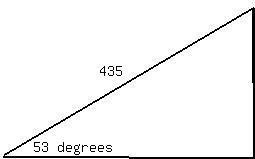# A rocket is launched at an angle of 53 degrees above the horizontal with an initial speed of 100 m/s. The rocket moves for 3.00 s a long its initial lcoexpennan 2021-02-13 Answered
A rocket is launched at an angle of 53 degrees above the horizontal with an initial speed of 100 m/s. The rocket moves for 3.00 s a long its initial line of motion with an acceleration of 30.0 m/s/s. At this time, its engines fail and the rocket proceeds to move as a projectile. Find:
a) the maximum altitude reached by the rocket
b) its total time of flight
c) its horizontal range.
You can still ask an expert for help

• Questions are typically answered in as fast as 30 minutes

Solve your problem for the price of one coffee

• Math expert for every subject
• Pay only if we can solve itoppturf

Lets find the maximum altitude of the rocket
Lets not worry about the components until the rocket has done accelerating.
The angle will remain constant during acceleration.
The velocity the second the rocket stops accelerating is
${V}_{f}=v+at$
${V}_{f}=100+30\left(3\right)$
${V}_{f}=190$m/s still acting at 53 degrees.
how far has it moved in each direction
$d=Vb+\frac{1}{2}$
d=100(3)+15(9)
d=435m
Lets look ar drawingSo we can do some basic trig to find its height and horizontal distance

now we have the height and distance when it stops accelerating. Now its a simple projectile motion question
Going back we found it had a velocity of 190 m/s
Lets split that into x and y component
Velocity $y=\mathrm{sin}53\cdot 190=151.7$ m/s
Velocity $x=\mathrm{cos}53\cdot 190=114.3$ m/s
a) Max altitude just concerned with y component now our old Vf is our new Vi when it reaches its maximum height the velocity final will be zero since gravity is accelerating it down
Vf=V+at
0=151.7-9.8t $t=\frac{-151.7}{-9.8}$ t=15.4s
Again
$d=Vb+\frac{1}{2}at$
$d=151.7\left(15.4\right)-4.9{\left(15.4\right)}^{2}$
d=2336m-1162m=1174
But that was after the engines remember before the engines blew it had a height of 357.4 m
so total height =1174+357.4=1531.6 m
b) so right now we know the total time to its max height but we still need to find the time it takes to free fall to earth
$d=Vb+\frac{1}{2}at$
${V}_{1}=0$ now
$d=4.9{t}^{2}$
${t}^{2}=\frac{1531.6}{4.9}=312.6$
t=17.6 s
so lets add up the total times
3 s till engine fall , 15.4 s till it reaches max alt and 17.6 seconds down
Total time = 36 s
c) horinzontal range
once the engines blew the x velocity was constant.
recall
Velocity $x=cdos53\cdot 190=114.3$ m/s
so d=t
d=114.3(33) the first 3 seconds werent constant
d=3771.9
but we must add on the original distance when engine blew
distance =261.7m
so total distance =3771.9+261.7=4033.6 m

###### Not exactly what you’re looking for?Jeffrey Jordon

Solution

Speed of the rocket after 3seconds =$100+3×3=190m/s$

displacement = $100×3+\frac{1}{2}×30×{3}^{2}=435m$

vertical displacement = $435\mathrm{sin}{53}^{\circ }=348m$

horizontal displacement = $435\mathrm{cos}{53}^{\circ }=261m$

$H=\frac{{u}_{y}^{2}}{2g}=649.8m$

max Altitude = 649.8 + 348 = 997.8 m

time of flight under gravity is give by

$-348=152T-\frac{1}{2}g{T}^{2}⇒T=32.5s$

total time of flight = 32.5 + 3 =35.5 s

horizontal range = $114×32.5+261=3966m$